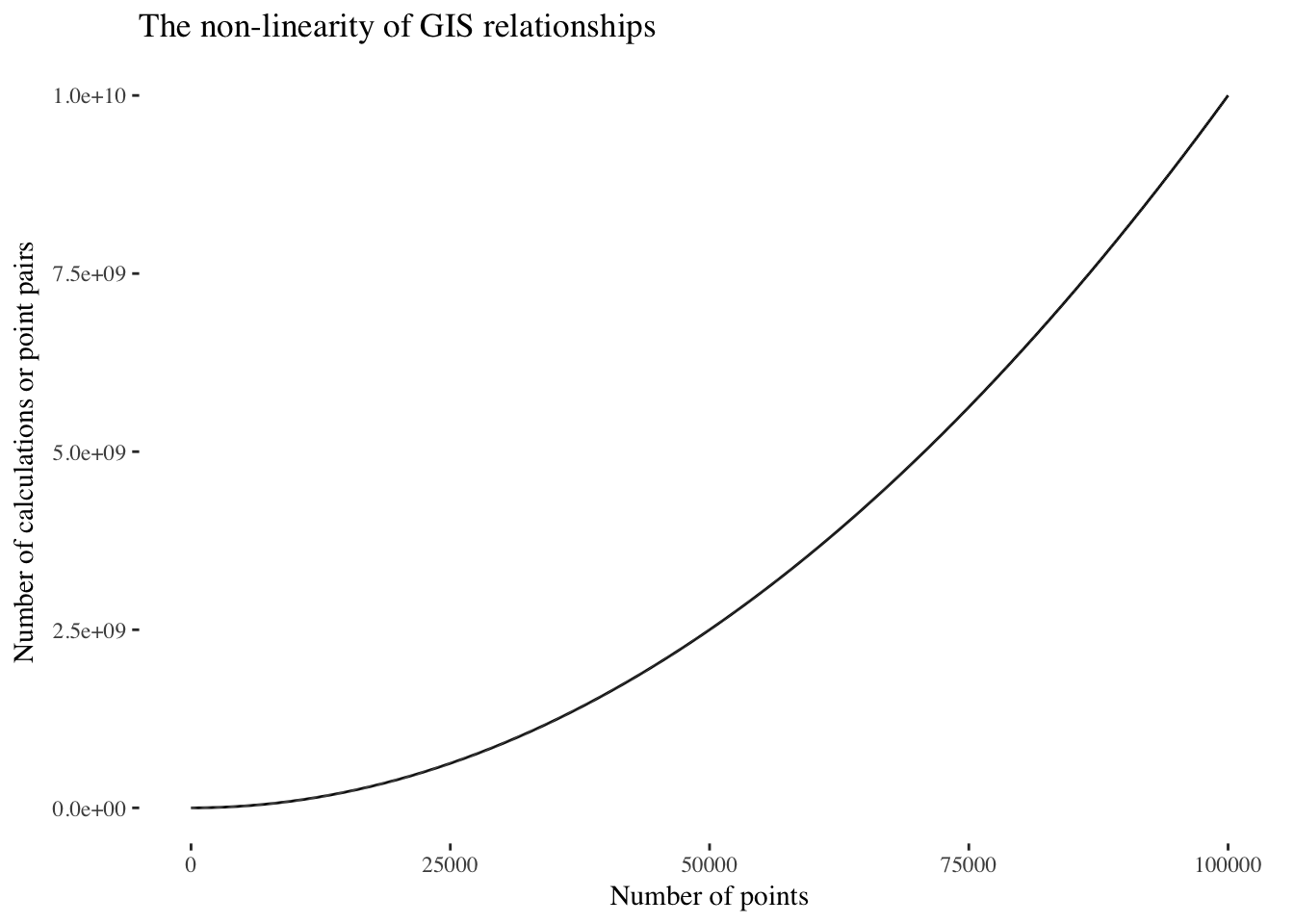# Spatial Analytics

Geography, some economics and data analysis.

# A function for parallelisation of spatial analysis in R

### Phil Donovan / 2017-09-11

In my previous post on parallising GIS workflows, I showed how you can parallise GIS analysis in R, and the potential gains from it. Long computation times are a common problem when working with spatial/GIS data. Inherently, most spatial relationships do not scale well. For example, calculating the distance to distance of a pair of GIS layers is an exponential relationship ($$x^x$$)1. The figure below illustrates this.This non-linearity in computation plays havoc on GIS practitioners lives as it makes it very hard to estimate how long it will take to perform an operation. Much to the vexation of their managers.

As discussed in my previous post, recent advancements in GIS functionality in R has made analysis even easier and quicker than ever. The brilliance of using R is that it provides a much more natural way of working with data, either spatial or non-spatial. And like Python, R comes with a more general suite of packages than the your bog standard GIS system such as ArcGIS; which means you can extend yourself much further than GIS if you need to. An example of this, is the parallel package which, when combined with the simple features package enables parallisation of GIS operations.

In my previous post, I showed how to use it. In this post I want to write a function which automatically parallises the analyses regardless of the GIS operation (e.g. st_intersects, st_distance, st_buffer etc). To do this I want a function which takes the following input:

1. a data set,
2. a simple features operation (st_intersects),
3. the number of cores to use, and
4. all other arguments passed to each unique st_ operation e.g. (buffer distances, or other simple features data set to intersect).

Below is the function I have come up with:

# Paralise any simple features analysis.
st_par <- function(sf_df, sf_func, n_cores, ...){

# Create a vector to split the data set up by.
split_vector <- rep(1:n_cores, each = nrow(sf_df) / n_cores, length.out = nrow(sf_df))

# Perform GIS analysis
split_results <- split(sf_df, split_vector) %>%
mclapply(function(x) sf_func(x, ...), mc.cores = n_cores)

# Combine results back together. Method of combining depends on the output from the function.
if ( class(split_results[]) == 'list' ){
result <- do.call("c", split_results)
names(result) <- NULL
} else {
result <- do.call("rbind", split_results)
}

# Return result
return(result)
}

This functions takes 1) a simple feature data frame, 2) a simple features function, 3) the number of cores to use, and 4) any number of extra arguments (...) which get passed into the specified simple features function.

Let’s generate some test data

sf_df_1 <- matrix(rnorm(12),nc=2) %>% data.frame %>% st_as_sf(coords = c("X1", "X2"))
sf_df_2 <- matrix(rnorm(12),nc=2) %>% data.frame %>% st_as_sf(coords = c("X1", "X2"))

and test this function.

distance_par <- st_par(sf_df_1, st_distance, 3, y = sf_df_2)
distance_par
##           [,1]      [,2]     [,3]     [,4]      [,5]      [,6]
## [1,] 0.9301662 1.4703934 1.872042 2.468495 0.8159693 0.7891409
## [2,] 0.8543219 0.6100950 1.705988 2.554656 1.0360668 0.5147192
## [3,] 0.9761547 1.4032367 1.930220 2.553768 0.8911063 0.6886363
## [4,] 1.7718170 1.1859306 2.699706 3.481833 1.8334608 0.5320917
## [5,] 1.7845450 1.8562116 1.326549 2.098716 2.0604793 2.5197328
## [6,] 1.8146996 0.9597656 2.689117 3.529525 1.9321772 0.6185279

OK, great! Is this exactly the same as the output from the normal, non-parallel analysis?

distance_normal <- st_distance(sf_df_1, sf_df_2)
identical(distance_normal, distance_par)
##  TRUE

This is exactly the output we expect. We are getting a matrix of the results which were processed in parallel exactly as we would if we’d run the function normally.

Next time, I’d like to make this function so that it works on Windows, using the parLapply instead of the mclapply2. It’s a pain, as it is less elegant than mclapply, but it would extend the functionality to everyone else which is important when it is clients!

1. e.g. the number of calculations between two data sets consisting of 10 points is $$10^{10}$$ or 100 in total.

2. mclapply only works on Linux and Mac OSX.